## 5.3Convergence

The Gauss-Seidel method was demonstrated in Sec. 5.2 using a sample problem, Eq. (5.2 ), that converged to within 0.2% accuracy in 9 solution sweeps. This section discusses the criteria for convergence of a solution.

The easiest explanation of convergence considers the error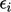for each equation, deﬁned in Sec. 5.2 . Substituting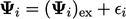in each term in Eq. (5.3 ), e.g. in Eq. (5.3a ), gives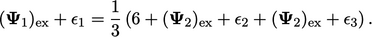(5.5)
Since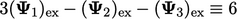, Eq. (5.5 ) reduces to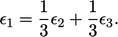(5.6)
The magnitude of error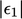is at least as large as the sum of the terms on the r.h.s., but is smaller if the signs of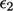and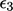are diﬀerent, i.e.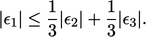(5.7)
Repeating for Eq. (5.3b ) and Eq. (5.3c ) gives: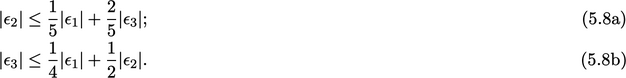The solution begins with an initial error of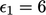,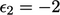and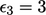. After one sweep the error is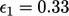,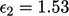and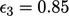.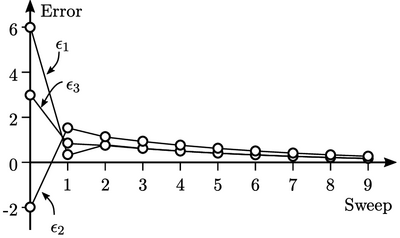The error is quickly distributed evenly, such that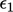and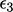are almost identical at sweep 2. The errors continue to reduce since Eq. (5.7 ) and Eq. (5.8 ) guarantee that no error is greater than the average of the other errors.

### Condition for convergence

The behaviour of this problem indicates a convergence condition for the Gauss-Seidel method: the magnitude of the diagonal coeﬃcient in each matrix row must be greater than or equal to the sum of the magnitudes of the other coeﬃcients in the row; in one row at least, the “greater than” condition must hold.

This is known as diagonal dominance, which is a suﬃcient condition for convergence, described mathematically as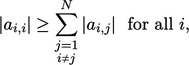(5.9)
where the ‘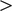’ condition must be satisﬁed for at least one. The description of the condition as “suﬃcient” means that convergence may occur when the condition is not met.
Notes on CFD: General Principles - 5.3 Convergence